# 编译原理（三）

正则表达式是一种用来描述正则语言的更紧凑的表示方法。正则表达式可以由较小的正则表达式按照特定的规则递归地构建。每个正则表达式r定义（表示）一个语言，记为L(r)。这个语言也是根据r的子表达式所表示的语言递归定义的。

r=a(a|b)*(ε|(.|_)(a|b)(a|b)*)

1.正则表达式的定义

ε是一个RE，L(ε)={ε};

a∈∑，则a是一个RE，L(a)={a}；

假设r和s都是RE，表示的语言分别是L(r)和L(s)，则

r|s是一个RE，L(r|s)=L(r)∪L(s)

rs是一个RE，L(rs)=L(r)L(s)

r*是一个RE，L(r*)=(L(r))*

(r)是一个RE，L((r))

运算符的优先级 ：*、连接、| 。

L(a|b)=L(a)L(b)={a}{b}={a,b}

L((a|b)(a|b))=L(a|b)L(a|b)={a,b}{a,b}={aa,ab,ba,bb}

L(a*)=(L(a))*={a}*={ε,a,aa,aaa,···}

L((a|b)*)=(L(a|b))*={a,b}*={ε,a,b,aa,ab,ba,bb,aaa,···}

L(a|a*b)={a,b,ab,aab,aaab,···}

2.正则语言

可以用RE定义的语言叫做正则语言或者正则集合。

3.正则文法与正则表达式

对任何正则文法G，存在定义同一语言的正则表达式r，对任何正则表达式r，存在定义同一语言的正则文法G。

4.正则定义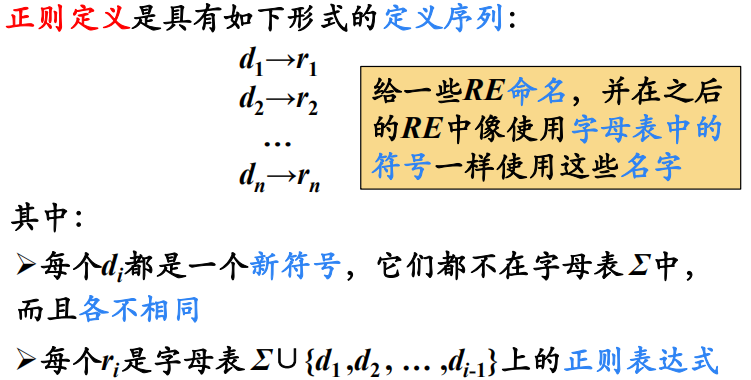digit→ 0|1|2|···|9

letter_ → A|B|···|Z|a|b|···|z|_

id→ letter_(letter_|digit*)

digit→ 0|1|2|···|9

digits→ digit digit*

optionalFraction → .digits| ε

optionalExponent → (E(+|-|ε )digits)|ε

number → digits  optionalExponent optionalFraction

2             2.15          2.15E+3          2.15E-3           2.15E3           2E-3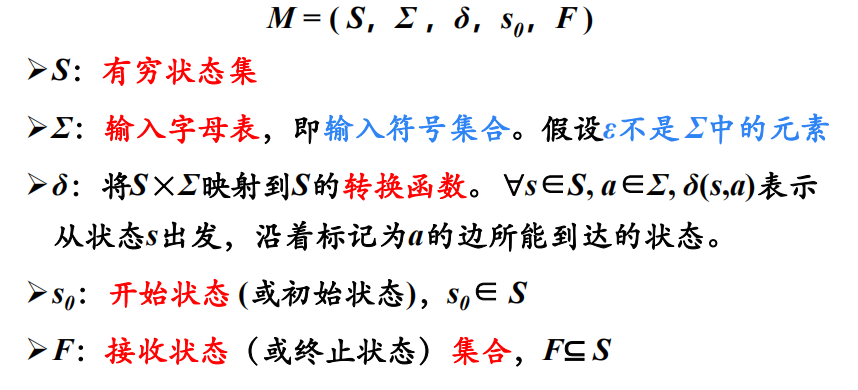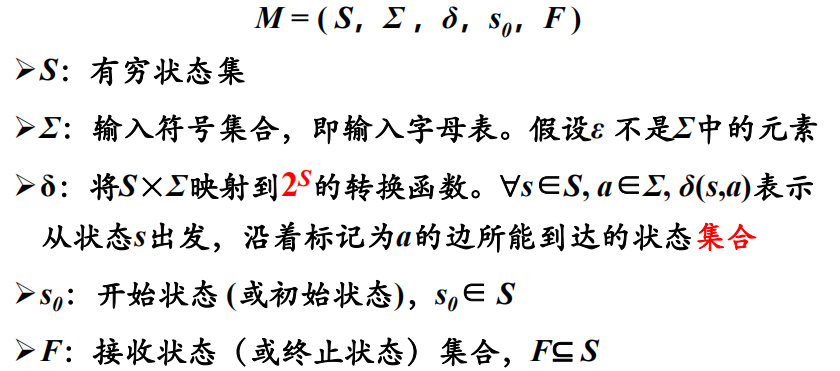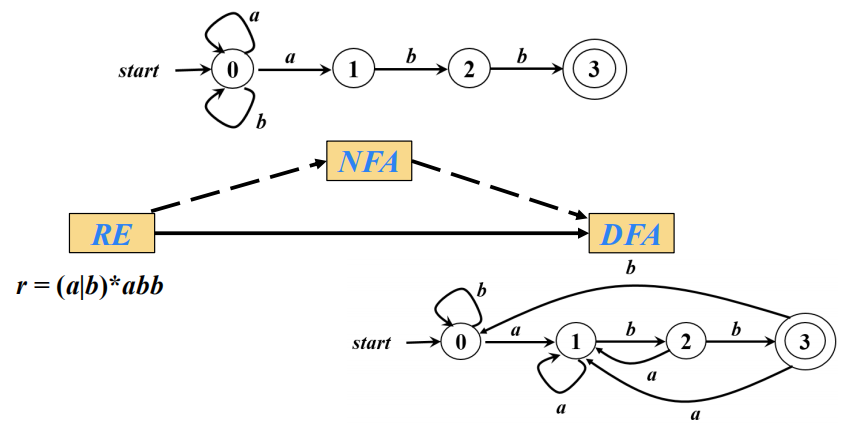1.RE转换NFA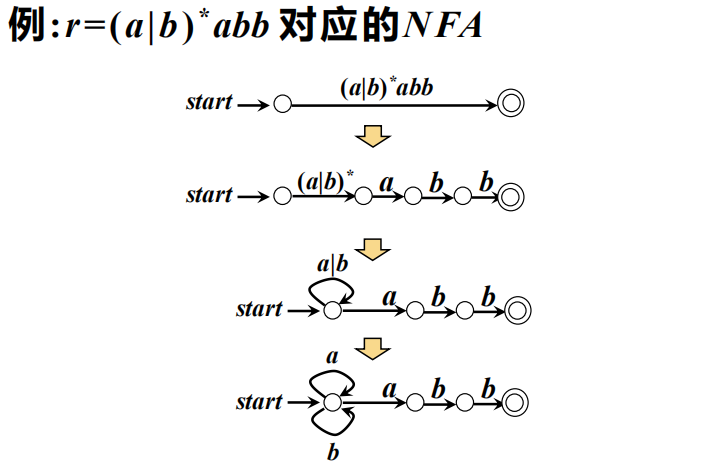2.NFA转换DFA（确定化）

子集构造法：NFA转换DFA的方法(状态转换矩阵)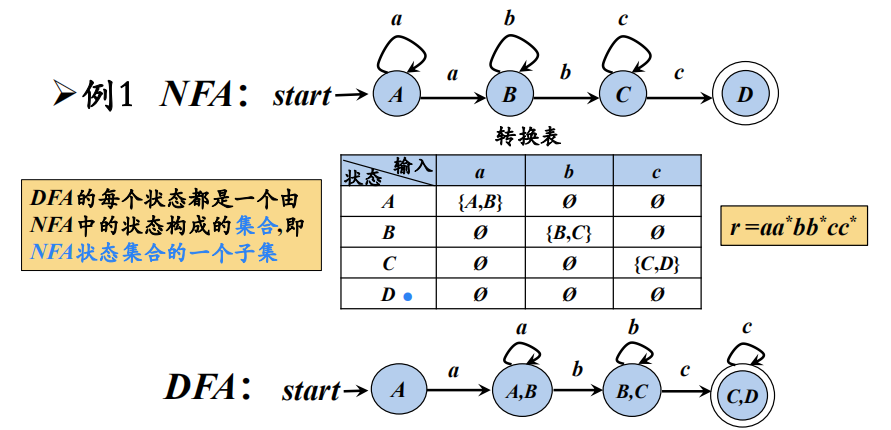状态集的ε-闭包：状态集I中的任何状态s及经任意条ε弧而能到达的所有状态的集合，定义为状态集I的ε-闭包，表示为ε-closure(I)。

状态集的a弧转换：状态集I中的任何状态s经过一条a弧而能到达的所有状态的集合，定义为状态集I的a弧转换，表示为move(I, a)。对于任意 NFA M=(K，Σ，f，S，F)，

I包含于K，a∈Σ，不妨设I={s1,s2,…sj }，则move(I,a)=f(s1,a)∪f(s2,a) ∪…∪f(sj,a)。

状态集的a弧转换的闭包：Ia=ε-closure(move(I,a))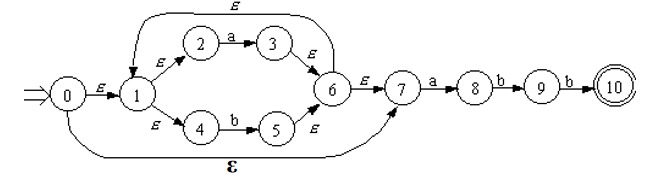对于I={0}，ε-closure(I)=ε-closure({0})={0,1,2,4,7}，

若I={2,3}，ε-closure(I)=ε-closure({2,3})={1,2,3,4,6,7}，

令I ={0,1,2,4,7}，则move(I,a)={3,8}，move(I,b)={5}，

Ia=ε-closure(move(I,a))=ε-closure({3,8})={1,2,3,4,6,7,8}

Ib=ε-closure(move(I,b))=ε-closure({5})={1,2,4,5,6,7}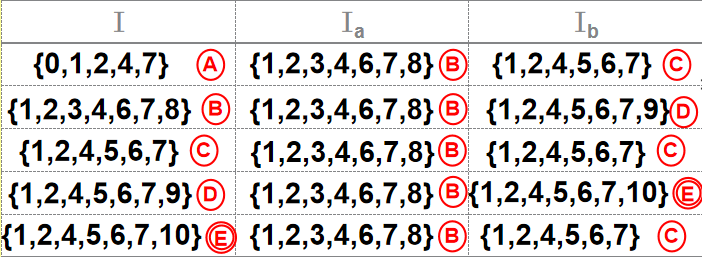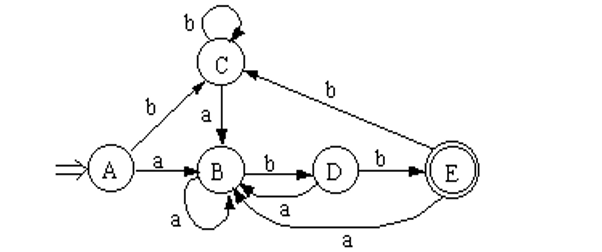3.DFA的最小化

对于任意一个DFA M构造另一个DFA M' ，使L(M)=L(M')，并且M'的状态个数不多于M的状态个数。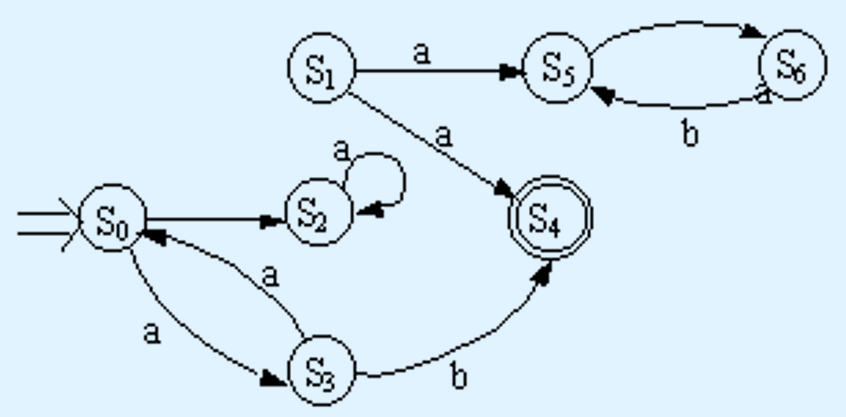多余状态：对于一个状态Si ，若从开始状态出发，不可能到达该状态Si，则Si为多余（无用）状态。 S1，S5，S6为多余状态。

死状态：对于一个状态Si，对任意输入符号a，若转到它本身后，不可能从它到达终止状态，则称为Si为死状态。S2为死状态。多余状态和死状态又称为无关状态。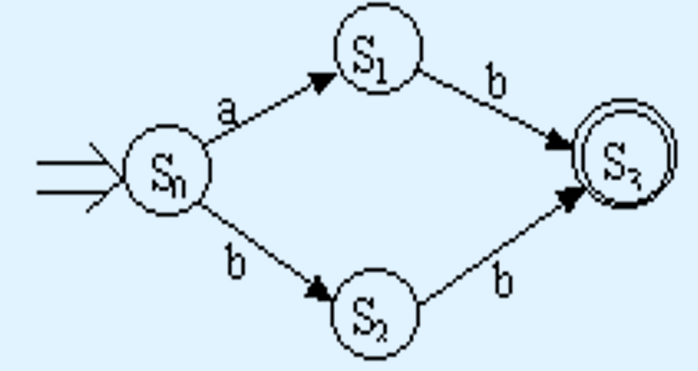等价状态：若Si为自动机的一个状态，我们把从Si出发能导出的所有符号串集合记为L(Si)。设有两个状态Si和Sj，若有L(Si)=L(Sj)，则称Si和Sj是等价状态。S1和S2是等价状态。

可区别状态：自动机中的两个状态Si和Sj，如果它们不等价，则称它们是可区别的。状态Si和Sj 必须同时是终止状态或同时是非终止状态，即终止状态和非终止状态是可区别的；状态Si和Sj对于任意输入符号a∈∑，必须转到等价的状态里，否则Si和Sj是可区别的。S0、S1、S2和S3是可区别的，S0和S2是可区别的。

DFA的最简化(最小化)的步骤：对于DFA M=(S,Σ,f,S0,Z)

1.首先将DFA的状态集进行初始化，分成π=(S-Z,Z);      //非终态、终态

2.用下面的过程对Π构造新的划分π new：

对Π中每个组G，G中的任意两个状态Si和Sj在同一组中，当且仅当对于Σ中任意输入符号 a ，Si和Sj的a转换是到同一组中，move(Si, a) ∈Gi ,move(Sj, a) ∈Gi。只要Si和Sj的a转换是到不同的组中，则说明Si和Sj是可区别的，可进行划分。

在Π new中用刚完成的对G的划分代替原来的G。

3.重复执行(2),直到Π中每个状态集不能再划分 （π new=π)为止;

4.合并等价状态,在每个G中,取任意状态作为代表,删去其它状态;

5.删去无关状态,从其它状态到无关状态的转换都成为无定义。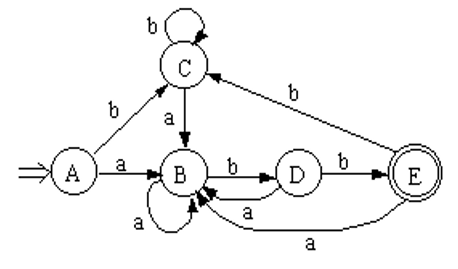第一步：首次划分，Π0=({A,B,C,D},{E})；

第二步：在G={A,B,C,D}中，f(A,a)=B，f(B,a)=B，f(C,a)=B，f(D,a)=B，f(A,b)=C，f(B,b)=D，f(C,b)=C，f(D,b)=E(终态)，故可以划分为{A,B,C}和{D}，Π1=({A,B,C},{D},{E})；

第三步：在G={A,B,C}中，f(A,a)=B，f(B,a)=B，f(C,a)=B，f(A,b)=C，f(B,b)=D，f(C,b)=C，故{A,B,C}可划分为{A,C}和{B}，Π2=({A,C},{B},{D},{E})；

第四步：在G={A,C}中，不可再分，则将A、C作为一个状态。

删去C，再将原来导入C的弧导入它的代表状态A。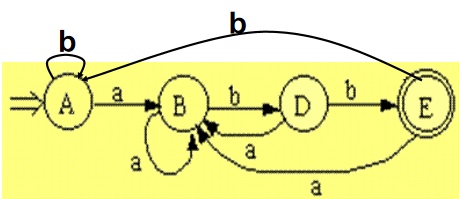逆波兰式（Reverse Polish notation，RPN，或逆波兰记法），也叫后缀表达式（将运算符写在操作数之后）

(a+b)*c-(a+b)/e  →((a+b)*c)((a+b)/e)-   →((a+b)c*)((a+b)e/)-   →(ab+c*)(ab+e/)-     →ab+c*ab+e/-

将一个普通的中序表达式转换为逆波兰表达式的一般算法是：

首先需要分配2个栈，一个作为临时存储运算符的栈S1（含一个结束符号），一个作为输入逆波兰式的栈S2（空栈），S1栈可先放入优先级最低的运算符#，注意，中缀式应以此最低优先级的运算符结束。可指定其他字符，不一定非#不可。从中缀式的左端开始取字符，逐序进行如下步骤：

（1）若取出的字符是操作数，则分析出完整的运算数，该操作数直接送入S2栈

（2）若取出的字符是运算符，则将该运算符与S1栈栈顶元素比较，如果该运算符优先级(不包括括号运算符)大于S1栈栈顶运算符优先级，则将该运算符进S1栈，否则，将S1栈的栈顶运算符弹出，送入S2栈中，直至S1栈栈顶运算符低于（不包括等于）该运算符优先级，最后将该运算符送入S1栈。

（3）若取出的字符是“（”，则直接送入S1栈顶。

（4）若取出的字符是“）”，则将距离S1栈栈顶最近的“（”之间的运算符，逐个出栈，依次送入S2栈，此时抛弃“（”。

（5）重复上面的1~4步，直至处理完所有的输入字符

（6）若取出的字符是“#”，则将S1栈内所有运算符（不包括“#”），逐个出栈，依次送入S2栈。©️2019 CSDN 皮肤主题: 数字20 设计师: CSDN官方博客